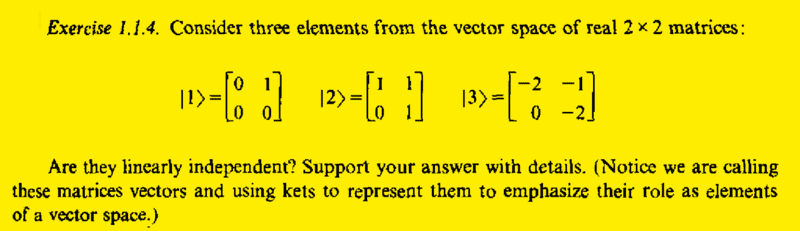# Checking the linear independence of elements of 2 X 2 matrices

• Pushoam
Thank you for catching that.In summary, the conversation discussed a problem involving linear independence and finding coefficients for a linear combination. One way to find the coefficients is by solving a system of equations, while another way is to use the definition of linear independence. The problem was ultimately solved by solving a reduced problem and checking the resulting values.f

## Homework Statement## Homework Equations

3. The Attempt at a Solution [/B]
## |3 \rangle = |1 \rangle - 2 ~ |2 \rangle ##
So, they are not linearly independent.

One way to find the coefficients is :
## |3 \rangle = a~ |1 \rangle +b~ |2 \rangle ## ...(1)
And solve (1) to get the values of a and b.

Is there any other easier way?

#### Attachments

Last edited:
One way to find the coefficients is :
## |3 \rangle = a~ |1 \rangle +b~ |2 \rangle ## ...(1)
And solve (1) to get the values of a and b.

Is there any other easier way?
It doesn't get much easier than that. Since ##a## and ##b## are overdetermined, you can choose two elements from each matrix (the right column from each, for example) and solve the reduced problem

##\begin{bmatrix} 1 & 1\\0 & 1 \end{bmatrix} \begin{bmatrix} a\\b \end{bmatrix} = \begin{bmatrix} -1\\-2 \end{bmatrix}##

and then check the resulting ##a## and ##b## on the left columns.

•Pushoam

## Homework Statement

View attachment 229238

## Homework Equations

3. The Attempt at a Solution [/B]
## |3 \rangle = |1 \rangle - 2 ~ |2 \rangle ##
So, they are not linearly independent.

One way to find the coefficients is :
## |3 \rangle = a~ |1 \rangle +b~ |2 \rangle ## ...(1)
And solve (1) to get the values of a and b.

Is there any other easier way?
I think you have done things the easiest way -- by inspection.

Another way is to use the definition of linear independence. The matrices A, B, and C (which you are calling |1>, |2>, and |3>) are linearly independent if there is only the nontrivial solution for the constants in the equation ##c_1A + c_2B + c_3C = 0##. By nontrivial, I mean solutions other than ##c_1 = c_2 = c_3 = 0##.

Last edited:
•Pushoam
It doesn't get much easier than that. Since ##a## and ##b## are overdetermined, you can choose two elements from each matrix (the right column from each, for example) and solve the reduced problem

##\begin{bmatrix} 1 & 1\\0 & 1 \end{bmatrix} \begin{bmatrix} a\\b \end{bmatrix} = \begin{bmatrix} -1\\-2 \end{bmatrix}##

and then check the resulting ##a## and ##b## on the left columns.
I did not understand what you meant by "##a## and ##b## are overdetermined". When to use this concept in general?

I did not understand what you meant by "##a## and ##b## are overdetermined". When to use this concept in general?
An overdetermined problem is one in which you have more equations than variables. In this particular case you have four linear equations to solve for two variables.
##0a+b=-2\\a+b=-1\\0a+0b=0\\0a+b=-2##
or
##\begin{bmatrix}0 &1\\1&1\\0&0\\0&1\end{bmatrix} \begin{bmatrix}a\\b\end {bmatrix}= \begin{bmatrix}-2\\-1\\0\\-2\end{bmatrix}##

In general such a system of equations can have no solutions, one solution, or an infinite number of solutions. There are no solutions if and only if the vectors are independent. You can use Gauss-Seidel to determine this. Or you can try to find a set of linearly independent rows (easy in this case) in the leftmost matrix, remove the other rows (on both sides), and solve the reduced problem.

Last edited by a moderator:
•Pushoam
An overdetermined problem is one in which you have more equations than variables. In this particular case you have four linear equations to solve for two variables.
##0a+b=-2\\a+b=-1\\0a+0b=0\\0a+b=-2##
or
##\begin{bmatrix}0 &1\\1&1\\0&0\\0&1\end{bmatrix} \begin{bmatrix}a\\b\end {bmatrix}= \begin{bmatrix}-2\\-1\\0\\-2\end{bmatrix}##

In general such a system of equations can have no solutions, one solution, or an infinite number of solutions. There are no solutions if and only if the vectors are independent. You can use Gauss-Seidel to determine this. Or you can try to find a set of linearly independent rows (easy in this case) in the leftmost matrix, remove the other rows (on both sides), and solve the reduced problem.
It is pretty easy in this case. The first equation says that ##0 a + b = -2##, so ##b = -2.## The second equation says that ##a+b = - 1\: \Rightarrow a = -1 - b = -1 + 2 = 1.## Now check if these values of ##a## and ##b## satisfy the third and fourth equations.

I think you have done things the easiest way -- by inspection.

Another way is to use the definition of linear independence. The matrices A, B, and C (which you are calling |1>, |2>, and |3>) are linearly independent if there are nontrivial solutions for the constants in the equation ##c_1A + c_2B + c_3C = 0##. By nontrivial, I mean solutions other than ##c_1 = c_2 = c_3 = 0##.
I think you probably wanted to write "linearly dependent " instead of "linearly independent".

I think you probably wanted to write "linearly dependent " instead of "linearly independent".
Yes, I did. I'll fix my original post.